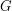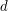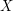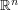## A map of the Tricki

This is an attempt to give a quick guide to the top few levels of the Tricki. It may cease to be feasible when the Tricki gets bigger, but we might perhaps be able to automate additions to it. Clicking on arrows just to the right of the name of an article reveals its subarticles. If you want to hide the subarticles again, then you should click to the right of them rather than clicking on the name of one of the subarticles themselves, since otherwise you will follow a link to that subarticle.

• Algebra
• Group theory
• Basic examples of groups Quick description (This page contains descriptions of a number of groups that can be used as tests for the truth or otherwise of general group-theoretic statements.)
• How to build groups
• Presentations of groups
• How to use group actions
• Proving results by letting a group act on a finite set Quick description (There are a number of group theoretic statements that do not mention group actions, but which can be solved by defining a set on which a given group acts and studying that action. Typically, the set is defined in terms of the group itself. This article gives several examples, and tips for choosing an appropriate action.)
• Representation theory
• Actions on topological spaces Quick description (If you want to study a group, try to realizeas the fundamental group of a topological space. This works best whenis infinite and discrete, especially ifis finitely presented and torsion-free.)
• Algebraic geometry
• Counting constants
• Dimension counting via incidence varieties Quick description (There are many situations in algebraic geometry in which one wishes to compute dimensions of various spaces, such as the space of all lines contained in a given surface in projective space. One approach to doing this is via so-called incidence varieties. An incidence variety is essentially the graph of a relation (for example, the collection of all ordered pairs of lines and surfaces of a given degree, where the line lies on the surface), but thought of itself as a variety. Since the incidence variety is a set of ordered pairs, it admits two natural projections, onto the first or second member of each ordered pair. Playing off the information that one obtains from considering these two projections, it is often possible to make non-trivial deductions.)
• How to compute the dimensions of the fibres of a map of varieties
• Use finite fieldsQuick description (If you have an algebraic problem about the complex numbers, it might be possible to solve it by solving a similar, but easier, problem about finite fields.)
• Analysis
• Areas of analysis
• Real analysis
• How to use the Bolzano-Weierstrass theorem Quick description ( The Bolzano-Weierstrass theorem asserts that every bounded sequence of real numbers has a convergent subsequence. More generally, it states that ifis a closed bounded subset ofthen every sequence inhas a subsequence that converges to a point in. This article is not so much about the statement, or its proof, but about how to use it in applications. If you come across a statement of a certain form (explained in the article), then the Bolzano-Weierstrass theorem may well be helpful. )
• Constructing exotic sets and functions using limiting arguments Quick description ( If you want to construct a set or function with a strange property (for instance, you might want a function that was continuous everywhere and differentiable nowhere) then a good way of doing so is often to define your object as a limit of a sequence of objects that exhibit the behaviour you want on smaller and smaller distance scales.)
• I have a problem to solve in real analysis Quick description ( This is a page that tries to understand what kind of problem you are trying to solve, so that it can take you to appropriate advice on the Tricki.)
• Metric spaces
• Topological spaces
• Complex analysis
• Harmonic analysis
• Partial differential equations
• Functional analysis
• Geometric measure theory
• Dynamics
• Analytic techniques
• Techniques for proving inequalities
• Statistics